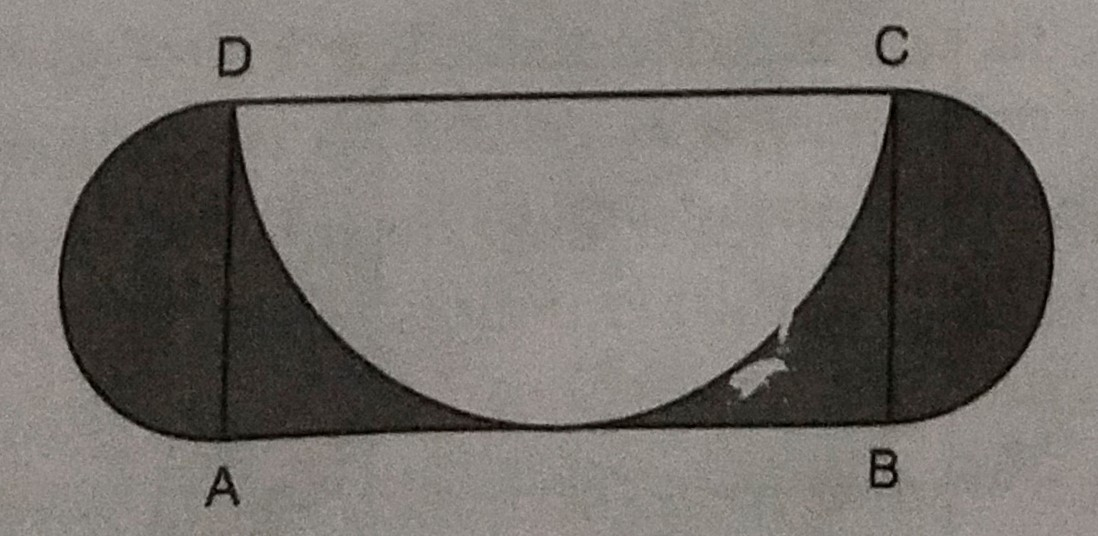"
">

# In the below figure, $A B C D$ is a rectangle with $A B=14 \mathrm{~cm}$ and $B C=7 \mathrm{~cm}$. Taking $D C, B C$ and $A D$ as diameters, three semi-circles are drawn as shown in the figure. Find the area of the shaded region."

Given:

$A B C D$ is a rectangle with $A B=14 \mathrm{~cm}$ and $B C=7 \mathrm{~cm}$.

To do:

We have to find the area of the shaded region.

Solution:

Two semicircles are drawn on $AD$ and $BC$ as diameters and third semicircle is drawn on $CD$ as diameter.

Area of the rectangle $ABCD = 14 \times 7 = 98\ cm^2$

Radius of the semicircle on $AD$ and $BC=\frac{\mathrm{AD}}{2}=\frac{7}{2} \mathrm{~cm}$

Area of two semicircles on $AD$ and $BC =2 \times \frac{1}{2} \pi r^{2}$

$=\frac{22}{7} \times \frac{7}{2} \times \frac{7}{2} \mathrm{~cm}^{2}$

$=\frac{77}{2} \mathrm{~cm}^{2}$

Radius of the semicircle on $CD=\frac{\mathrm{CD}}{2}=\frac{14}{2}=7 \mathrm{~cm}$

Area of the semicircle with CD as diameter $=\frac{1}{2} \pi \mathrm{R}^{2}$

$=\frac{1}{2} \times \frac{22}{7} \times 7 \times 7 \mathrm{~cm}^{2}$

$=77 \mathrm{~cm}^{2}$

Therefore,

Area of the shaded portion $=$ Area of rectangle $+$ Areas of two semicircles $-$ Area of the third semicircle

$=98+\frac{77}{2}-77$

$=(21+38.5) \mathrm{cm}^{2}$

$=59.5 \mathrm{~cm}^{2}$

The area of the shaded region is $59.5\ cm^2$.

Updated on: 10-Oct-2022

45 Views